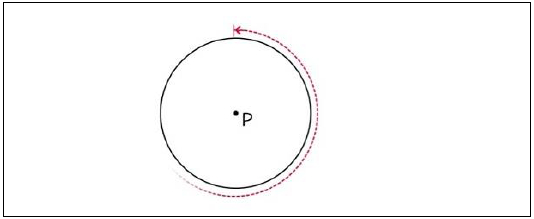# Java Program to Find the Perimeter of a Circle

In this article, we will understand how to find the perimeter of a circle. The circumference is the perimeter of a circle. It's the distance around a circle.

Circumference is given by the formula C = 2𝜋\pi r where, \pi𝜋 = 3.14 and r is the radius of the circle −Below is a demonstration of the same −

Input

Suppose our input is −

Radius of the circle : 5

Output

The desired output would be −

Perimeter of Circle is: 31.428571428571427


## Algorithm

Step 1 - START
Step 2 - Declare 2 double values namely my_radius and my_perimeter
Step 3 - Read the required values from the user/ define the values
Step 4 – Calculate the perimeter using the formula using the formula (2*22*7)/7 and store
the result.
Step 5- Display the result
Step 6- Stop

## Example 1

Here, the input is being entered by the user based on a prompt. You can try this example live in ourcoding ground tool.

import java.util.Scanner;
public class PerimeterOfCircle{
public static void main(String args[]){
System.out.println("Required packages have been imported");
Scanner my_scanner = new Scanner(System.in);
System.out.println("A reader object has been defined ");
System.out.print("Enter the radius of the circle : ");
System.out.println("The perimeter of Circle is: " +my_perimeter);
}
}

## Output

Required packages have been imported
A reader object has been defined
Enter the radius of the circle : 5
The perimeter of Circle is: 31.428571428571427

## Example 2

Here, the integer has been previously defined, and its value is accessed and displayed on the console.

public class PerimeterOfCircle{
public static void main(String args[]){
}
The radius of the circle is defined as 5.0
The perimeter of Circle is: 31.428571428571427